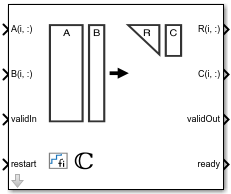Complex Burst QR Decomposition

QR decomposition for complex-valued matrices

• Library:
• Fixed-Point Designer HDL Support / Matrices and Linear Algebra / Matrix Factorizations

•Description

The Complex Burst QR Decomposition block uses QR decomposition to compute R and C = Q'B, where QR = A, and A and B are complex-valued matrices. The least-squares solution to Ax = B is x = R\C. R is an upper triangular matrix and Q is an orthogonal matrix. To compute C = Q', set B to be the identity matrix.

Ports

Input

expand all

Rows of matrix A, specified as a vector. A is an m-by-n matrix where m ≥ 2 and n ≥ 2. If B is single or double, A must be the same data type as B. If A is a fixed-point data type, A must be signed, use binary-point scaling, and have the same word length as B. Slope-bias representation is not supported for fixed-point data types.

Data Types: single | double | fixed point
Complex Number Support: Yes

Rows of matrix B, specified as a vector. B is an m-by-p matrix where m ≥ 2. If A is single or double, B must be the same data type as A. If B is a fixed-point data type, B must be signed, use binary-point scaling, and have the same word length as A. Slope-bias representation is not supported for fixed-point data types.

Data Types: single | double | fixed point
Complex Number Support: Yes

Whether inputs are valid, specified as a Boolean scalar. This control signal indicates when the data from the A(i,:) and B(i,:) input ports are valid. When this value is 1 (true) and the value at ready is 1 (true), the block captures the values on the A(i,:) and B(i,:) input ports. When this value is 0 (false), the block ignores the input samples.

After sending a true validIn signal, there may be some delay before ready is set to false. To ensure all data is processed, you must wait until ready is set to false before sending another true validIn signal.

Data Types: Boolean

Whether to clear internal states, specified as a Boolean scalar. When this value is 1 (true), the block stops the current calculation and clears all internal states. When this value is 0 (false), and the validIn value is 1 (true), the block begins a new subframe.

Data Types: Boolean

Output

expand all

Rows of the economy size QR decomposition matrix R, returned as a scalar or vector. R is an upper triangular matrix. R has the same data type as A.

Data Types: single | double | fixed point

Rows of the economy size QR decomposition matrix C=Q'B, returned as a scalar or vector. C has the same number of rows as R. C has the same data type as B.

Data Types: single | double | fixed point

Whether the output data is valid, returned as a Boolean scalar. This control signal indicates when the data at output ports R(i,:) and C(i,:) is valid. When this value is 1 (true), the block has successfully computed the R and C matrices. When this value is 0 (false), the output data is not valid.

Data Types: Boolean

Whether the block is ready, returned as a Boolean scalar. This control signal indicates when the block is ready for new input data. When this value is 1 (true), and the validIn value is 1 (true), the block accepts input data in the next time step. When this value is 0 (false), the block ignores input data in the next time step.

After sending a true validIn signal, there may be some delay before ready is set to false. To ensure all data is processed, you must wait until ready is set to false before sending another true validIn signal.

Data Types: Boolean

Parameters

expand all

The number of rows in matrices A and B, specified as a positive integer-valued scalar.

Programmatic Use

 Block Parameter: m Type: character vector Values: positive integer-valued scalar Default: 4

The number of columns in input matrix A, specified as a positive integer-valued scalar.

Programmatic Use

 Block Parameter: n Type: character vector Values: positive integer-valued scalar Default: 4

The number of columns in input matrix B, specified as a positive integer-valued scalar.

Programmatic Use

 Block Parameter: p Type: character vector Values: positive integer-valued scalar Default: 1

Tips

Use fixed.getQRDecompositionModel(A,B) to generate a template model containing a Complex Burst QR Decomposition block for complex-valued input matrices A and B.

Extended Capabilities

Introduced in R2019b

Fixed-Point Designer DocumentationGet trial now